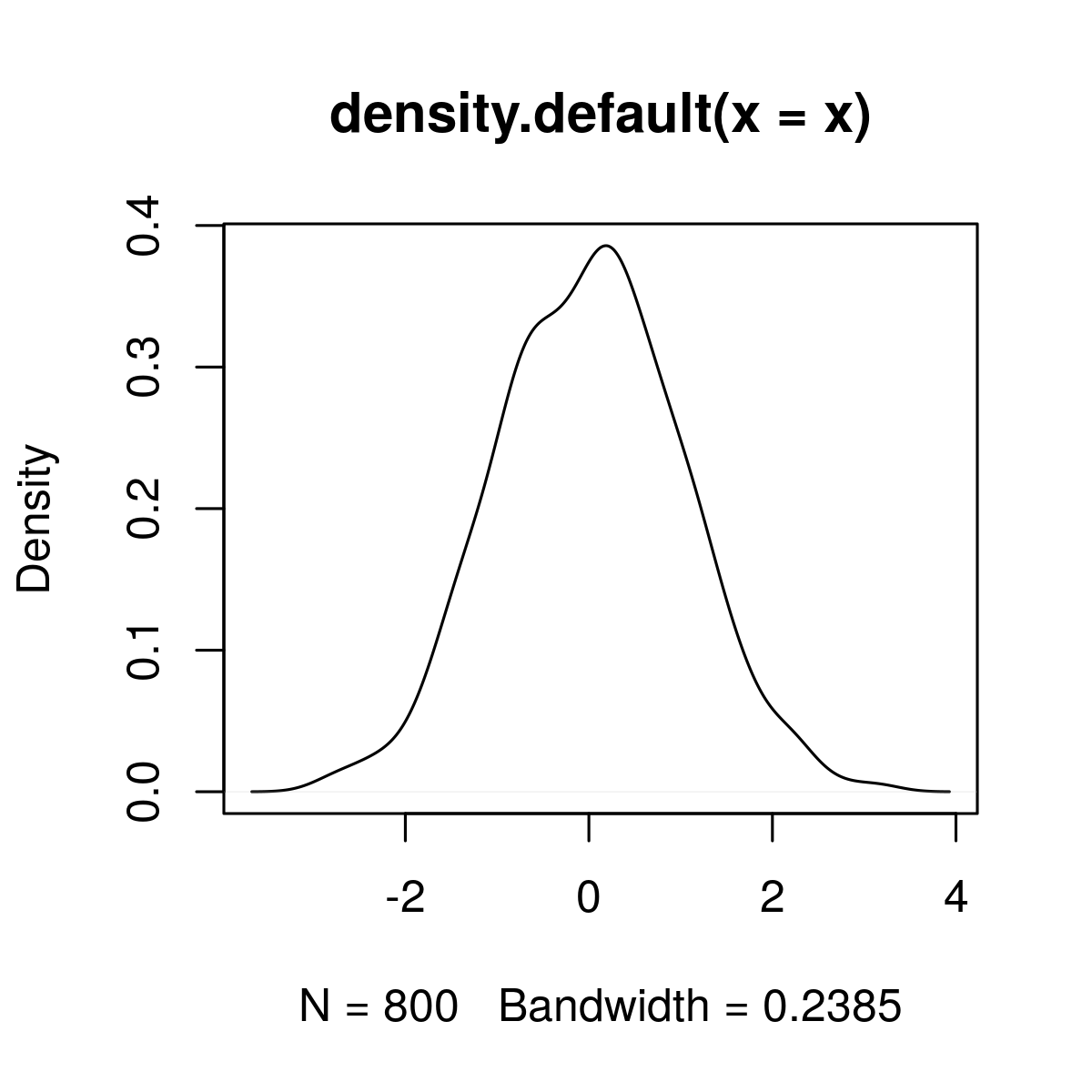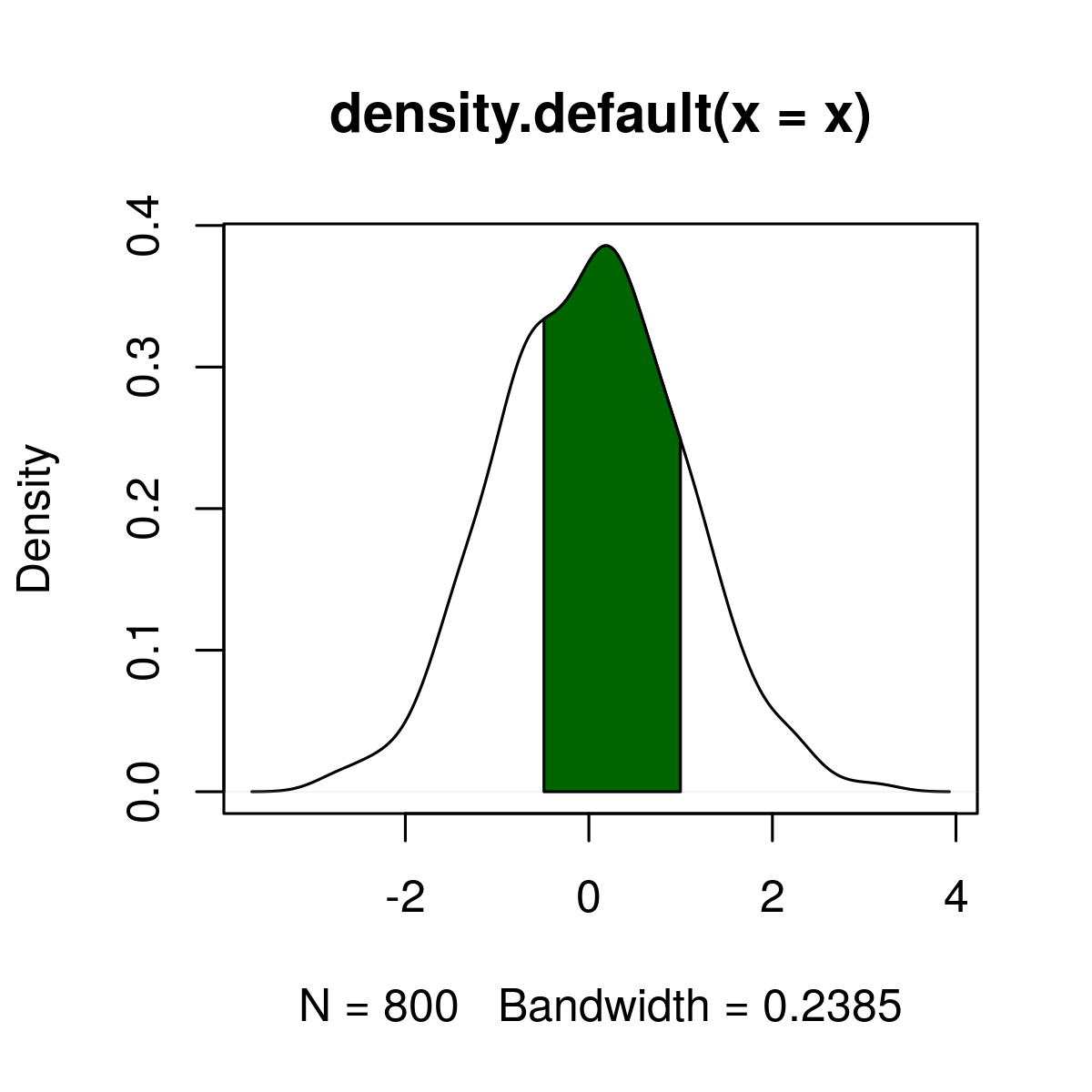# Add Color Between Two Points of Kernel Density Plot in R Programming – Using with() Function

plot() and with() functions are used to draw and add color between a particular area of two points of Kernel Density Plot in R Language.

#### Draw a Kernel Density plot

`plot()` function in R Language is used to draw a Kerner Density Plot

Syntax: plot(dens_x)

Parameters:
dens_x: Default plot of density.

Returns: Kernel density plot

 `# Create example data ` ` `  `set.seed``(72500)   ` `x <- ``rnorm``(800) ` `dens_x <- ``density``(x) ` `plot``(dens_x)        `

Output:`with()` function is used to add color between two points of the graph.

Syntax:
with(dens_x,
polygon(x = c(x),
y = c(0, y[x_low:x_high], 0),
col = ” “))

Parameters:
dens_x: Default plot of density.
x_low, x_high: lower limit and upper limit of colored area
col: Name of color

Returns: Color plot between to particular plots with specified color.

 `# color the plot ` ` `  `set.seed``(72500)   ` `x <- ``rnorm``(800) ` `dens_x <- ``density``(x) ` `plot``(dens_x)        ` `x_low <- ``min``(``which``(dens_x\$x >= - 0.5))   ``# Define lower limit of colored area ` `x_high <- ``max``(``which``(dens_x\$x < 1))       ``# Define upper limit of colored area ` `with``(dens_x,                             ``# Add color between two limits ` `     ``polygon``(x = ``c``(x[``c``(x_low, x_low:x_high, x_high)]), ` `             ``y = ``c``(0, y[x_low:x_high], 0), ` `             ``col = ``"darkgreen"``)) `

Output:Whether you're preparing for your first job interview or aiming to upskill in this ever-evolving tech landscape, GeeksforGeeks Courses are your key to success. We provide top-quality content at affordable prices, all geared towards accelerating your growth in a time-bound manner. Join the millions we've already empowered, and we're here to do the same for you. Don't miss out - check it out now!

Previous
Next